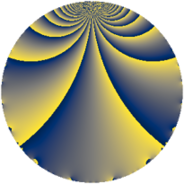# Properties

 Label 3920.1.j.aLevel $3920$ Weight $1$ Character orbit 3920.j Self dual yes Analytic conductor $1.956$ Analytic rank $0$ Dimension $1$ Projective image $D_{2}$ CM/RM discs -4, -20, 5 Inner twists $4$

# Learn more

## Newspace parameters

 Level: $$N$$ $$=$$ $$3920 = 2^{4} \cdot 5 \cdot 7^{2}$$ Weight: $$k$$ $$=$$ $$1$$ Character orbit: $$[\chi]$$ $$=$$ 3920.j (of order $$2$$, degree $$1$$, minimal)

## Newform invariants

 Self dual: yes Analytic conductor: $$1.95633484952$$ Analytic rank: $$0$$ Dimension: $$1$$ Coefficient field: $$\mathbb{Q}$$ Coefficient ring: $$\mathbb{Z}$$ Coefficient ring index: $$1$$ Twist minimal: no (minimal twist has level 80) Projective image: $$D_{2}$$ Projective field: Galois closure of $$\Q(i, \sqrt{5})$$ Artin image: $D_4$ Artin field: Galois closure of 4.0.15680.1

## $q$-expansion

 $$f(q)$$ $$=$$ $$q + q^{5} - q^{9} + O(q^{10})$$ $$q + q^{5} - q^{9} + q^{25} + 2q^{29} + 2q^{41} - q^{45} + 2q^{61} + q^{81} - 2q^{89} + O(q^{100})$$

## Character values

We give the values of $$\chi$$ on generators for $$\left(\mathbb{Z}/3920\mathbb{Z}\right)^\times$$.

 $$n$$ $$981$$ $$1471$$ $$3041$$ $$3137$$ $$\chi(n)$$ $$1$$ $$-1$$ $$1$$ $$-1$$

## Embeddings

For each embedding $$\iota_m$$ of the coefficient field, the values $$\iota_m(a_n)$$ are shown below.

For more information on an embedded modular form you can click on its label.

Label $$\iota_m(\nu)$$ $$a_{2}$$ $$a_{3}$$ $$a_{4}$$ $$a_{5}$$ $$a_{6}$$ $$a_{7}$$ $$a_{8}$$ $$a_{9}$$ $$a_{10}$$
3039.1
 0
0 0 0 1.00000 0 0 0 −1.00000 0
 $$n$$: e.g. 2-40 or 990-1000 Significant digits: Format: Complex embeddings Normalized embeddings Satake parameters Satake angles

## Inner twists

Char Parity Ord Mult Type
1.a even 1 1 trivial
4.b odd 2 1 CM by $$\Q(\sqrt{-1})$$
5.b even 2 1 RM by $$\Q(\sqrt{5})$$
20.d odd 2 1 CM by $$\Q(\sqrt{-5})$$

## Twists

By twisting character orbit
Char Parity Ord Mult Type Twist Min Dim
1.a even 1 1 trivial 3920.1.j.a 1
4.b odd 2 1 CM 3920.1.j.a 1
5.b even 2 1 RM 3920.1.j.a 1
7.b odd 2 1 80.1.h.a 1
7.c even 3 2 3920.1.bt.a 2
7.d odd 6 2 3920.1.bt.b 2
20.d odd 2 1 CM 3920.1.j.a 1
21.c even 2 1 720.1.j.a 1
28.d even 2 1 80.1.h.a 1
28.f even 6 2 3920.1.bt.b 2
28.g odd 6 2 3920.1.bt.a 2
35.c odd 2 1 80.1.h.a 1
35.f even 4 2 400.1.b.a 1
35.i odd 6 2 3920.1.bt.b 2
35.j even 6 2 3920.1.bt.a 2
56.e even 2 1 320.1.h.a 1
56.h odd 2 1 320.1.h.a 1
84.h odd 2 1 720.1.j.a 1
105.g even 2 1 720.1.j.a 1
105.k odd 4 2 3600.1.e.a 1
112.j even 4 2 1280.1.e.a 2
112.l odd 4 2 1280.1.e.a 2
140.c even 2 1 80.1.h.a 1
140.j odd 4 2 400.1.b.a 1
140.p odd 6 2 3920.1.bt.a 2
140.s even 6 2 3920.1.bt.b 2
168.e odd 2 1 2880.1.j.a 1
168.i even 2 1 2880.1.j.a 1
280.c odd 2 1 320.1.h.a 1
280.n even 2 1 320.1.h.a 1
280.s even 4 2 1600.1.b.a 1
280.y odd 4 2 1600.1.b.a 1
420.o odd 2 1 720.1.j.a 1
420.w even 4 2 3600.1.e.a 1
560.be even 4 2 1280.1.e.a 2
560.bf odd 4 2 1280.1.e.a 2
840.b odd 2 1 2880.1.j.a 1
840.u even 2 1 2880.1.j.a 1

By twisted newform orbit
Twist Min Dim Char Parity Ord Mult Type
80.1.h.a 1 7.b odd 2 1
80.1.h.a 1 28.d even 2 1
80.1.h.a 1 35.c odd 2 1
80.1.h.a 1 140.c even 2 1
320.1.h.a 1 56.e even 2 1
320.1.h.a 1 56.h odd 2 1
320.1.h.a 1 280.c odd 2 1
320.1.h.a 1 280.n even 2 1
400.1.b.a 1 35.f even 4 2
400.1.b.a 1 140.j odd 4 2
720.1.j.a 1 21.c even 2 1
720.1.j.a 1 84.h odd 2 1
720.1.j.a 1 105.g even 2 1
720.1.j.a 1 420.o odd 2 1
1280.1.e.a 2 112.j even 4 2
1280.1.e.a 2 112.l odd 4 2
1280.1.e.a 2 560.be even 4 2
1280.1.e.a 2 560.bf odd 4 2
1600.1.b.a 1 280.s even 4 2
1600.1.b.a 1 280.y odd 4 2
2880.1.j.a 1 168.e odd 2 1
2880.1.j.a 1 168.i even 2 1
2880.1.j.a 1 840.b odd 2 1
2880.1.j.a 1 840.u even 2 1
3600.1.e.a 1 105.k odd 4 2
3600.1.e.a 1 420.w even 4 2
3920.1.j.a 1 1.a even 1 1 trivial
3920.1.j.a 1 4.b odd 2 1 CM
3920.1.j.a 1 5.b even 2 1 RM
3920.1.j.a 1 20.d odd 2 1 CM
3920.1.bt.a 2 7.c even 3 2
3920.1.bt.a 2 28.g odd 6 2
3920.1.bt.a 2 35.j even 6 2
3920.1.bt.a 2 140.p odd 6 2
3920.1.bt.b 2 7.d odd 6 2
3920.1.bt.b 2 28.f even 6 2
3920.1.bt.b 2 35.i odd 6 2
3920.1.bt.b 2 140.s even 6 2

## Hecke kernels

This newform subspace can be constructed as the intersection of the kernels of the following linear operators acting on $$S_{1}^{\mathrm{new}}(3920, [\chi])$$:

 $$T_{3}$$ $$T_{11}$$ $$T_{13}$$ $$T_{41} - 2$$

## Hecke characteristic polynomials

$p$ $F_p(T)$
$2$ $$T$$
$3$ $$T$$
$5$ $$-1 + T$$
$7$ $$T$$
$11$ $$T$$
$13$ $$T$$
$17$ $$T$$
$19$ $$T$$
$23$ $$T$$
$29$ $$-2 + T$$
$31$ $$T$$
$37$ $$T$$
$41$ $$-2 + T$$
$43$ $$T$$
$47$ $$T$$
$53$ $$T$$
$59$ $$T$$
$61$ $$-2 + T$$
$67$ $$T$$
$71$ $$T$$
$73$ $$T$$
$79$ $$T$$
$83$ $$T$$
$89$ $$2 + T$$
$97$ $$T$$
show more
show less